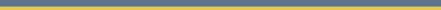Analysis of Economics Data: Further Courses

What courses to take after Economics 102 or similar regression course?
This is changing over time as there are more offerings.

FOR WHICH REGRESSION COURSE SHOULD I TAKE (ECN 102 or 140)
see  whichregressioncourse.html

ECONOMICS COURSES
• Economics 140 (Econometrics) This presents regression theory and methods in more detail than 102.
In 2021-22 this is offered in Fall 2021 and twice in Spring 2022.
• Economics 141 (Economics and Financial Forecasting) and requires ECN 102 or equivalent.
In 2021-22 this is offered in Fall 2021.
• Economics 190 (Topics in Economics - Economics and Business Data Analytics) requires ECN 102 or equivalent.
In 2021-22 this is offered in Spring 2022.
See http://www.econ.ucdavis.edu/courses/ecn-190-topics.
The 190 course will become regularly listed course (Economics 142) once approved by the university course approval committee.
• Several economics courses include some regression analysis of data.
These courses are in the Data Analytics and Economics Analysis Specialization Track of the Economics major.
Currently ECN 132 (Health Economics) and ECN 145 (Transportation Economics), ECN 151B (Economics of Human Resources), ECN 152 (Economics of Education) and some of the ECN 190 courses. More courses may be added over time.
STATISTICS COURSES
Statistics courses are listed here. Most immediately useful for economists are
• Statistics 108 (Applied Statistical Methods: Regression Analysis).
• Statistics 130A-B (Mathematical Statistics: Brief Course).
• Statistics 131A-C (Mathematical Statistics). More advanced version of 130A-B. Take either 130 or 131.
• Statistics 137 (Applied Time Series Analysis).
• Statistics 141A-C (Data Science, Data and Web Technologies, Big Data)
• Statistics 142A-B (Statistical Learning)
A minor in Statistics is Stat 106, 108, 130A-B (or 131A-B) and 1 course with 130B as a pre-requisite.
A major in Statistics requires 48-52 upper division units depending on which trak you take.

DATA STUDIES COURSES
This new small program offers two courses listed here.
• STS 101 (Introduction to Data Studies). Data manipulation using Excel.
• STS 112 (Visualizing Society with Data). Uses R.
• STS 115 (Data Science and Exploration). Case studies using R.
• STS 195 (Research in data Studies).
COMPUTER SCIENCE COURSES

The most useful first course for computer science non-majors is ECS 32A Introduction to Programming using Python. This is followed by ECS 32B and ECS 34. (Majors instead begin with ECS 36A which uses C++).

Computer science courses are listed here. The new numbering, beginning in 2018-19 follows. The lower division courses for non-majors  include
• ECS 32A (Introduction to Programming). Programming in Python (was ECS10).
• ECS 32B (Introduction to Data Structures) (was ECS60) uses Python.
• ECS 32C (Implementation of Data Structures in C). Uses C.
• ECS 34 (Software development in Unix and C/C++ (was ECS 40)
The lower division courses for majors include
• ECS 36A Programming and problem solving). Programming in C. (was ECS 30)
• ECS 36B Software Development and Object-oriented programming in C++ (was ECS 40)
• ECS 36C Data Structures, Algorithms and Programming (was ECS 60)
MATHEMATICS COURSES

Mathematics courses are listed here with detailed syllabi here.
To strengthen mathematics for economics take more advanced courses in linear algebra and calculus.
• MAT 22A (Linear Algebra). Covers vectors and matrices.
• MAT 67 (Modern Linear Algebra). More advanced course than 22A. Requires 21A.
• MAT 25 (Advanced Calculus). Requires 21C.
• MAT 127A (Real Analysis). Requires 25. Calculus at an even more advanced level
• MAT 108 (Introduction to Abstract mathematics). Requires 21B. Includes how to prove theorems .A. Colin Cameron / UC-Davis Economics / http://www.econ.ucdavis.edu/faculty/cameron